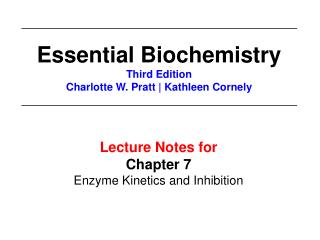# Lecture Notes for Chapter 7 Enzyme Kinetics and Inhibition - PowerPoint PPT PresentationDownload PresentationLecture Notes for Chapter 7 Enzyme Kinetics and Inhibition

Lecture Notes for Chapter 7 Enzyme Kinetics and InhibitionDownload Presentation## Lecture Notes for Chapter 7 Enzyme Kinetics and Inhibition

- - - - - - - - - - - - - - - - - - - - - - - - - - - E N D - - - - - - - - - - - - - - - - - - - - - - - - - - -
##### Presentation Transcript

1. Essential Biochemistry Third Edition Charlotte W. Pratt | Kathleen Cornely Lecture Notes for Chapter 7 Enzyme Kinetics and Inhibition

2. KEY CONCEPTS: Section 7-1 • An enzyme’s activity, measured as the rate of product formation, varies with the substrate concentration.

3. Rates of Chemical Reactions • Enzyme kinetics is the study of rates of reactions catalyzed by enzymes. k v= S P • The reaction rate (velocity, v) can be described in several ways. • Disappearance of substrate, S • Appearance of product, P • These equations relate velocity to concentration of reactants and products.

4. Consider the reaction catalyzed by triose phosphate isomerase. • As [GAP] decreases, the [DHAP] increases. GAP DHAP

5. Many enzymes react with substrates in a nonlinear fashion. • The shape here is hyperbolic. • Shape indicates, in part, that E and S combine to form an ES complex E + S ES

6. KEY CONCEPTS: Section 7-2 • Simple chemical reactions are described in terms of rate constants. • The Michaelis-Menten equation describes enzyme-catalyzed reactions in terms of KM and Vmax.

7. Rate equations describe chemical processes. • A unimolecular reaction has a velocity (rate) that is dependent on the concentration of only one substrate. • v = k [A], where k has units of sec-1 k v= A P

8. Rate equations describe chemical processes. • A bimolecular (second order) reaction has a velocity (rate) that is dependent on two substrate concentrations. • v = k [A] [B] (or k [A]2 or k [B]2)where k has units of M-1 sec-1 k v= A + B P

9. k1 k2 E + S ES E + P k-1 Many enzymes obey Michaelis-Menten kinetics. Rate limiting step Problem: [ES] is difficult to measure!What can we do?

10. k1 k2 E + S ES E + P k-1 Try to re-express the rate. One point that helps: k1 [E] [S] - k-1 [ES] - k2 [ES] Formation of ES Depletion of ES

11. Assume steady state equilibrium. • For most of the duration of the reaction, [ES] remains steady as substrate is converted to product.

12. k1 k2 E + S ES E + P k-1 Michaelis-Menten Equation = k1 [E] [S] - k-1 [ES] - k2 [ES]

13. Derivation of the Michaelis-Menten Equation = k1 [E] [S] - k-1 [ES] - k2 [ES] Thus: k1 [E] [S] = [ES] (k-1 + k2 ) Since… [E]total = [ES] + [E] [E] = [E]total - [ES] Substitute here

14. Derivation of the Michaelis-Menten Equation k1 ([E]total [S] - [ES] [S]) = [ES] (k-1 + k2 ) KM Divide both sides by k1 [E]total [S] - [ES] [S] = [ES] (k-1 + k2 ) k1 [E]total [S] - [ES] [S] = [ES] KM

15. Derivation of the Michaelis-Menten Equation [E]total [S] - [ES] [S] = [ES] KM Rearrange: [E]total [S] = [ES] (KM + [S]) [ES] = [E]total [S] KM + [S]

16. Derivation of the Michaelis-Menten Equation Vmax k2 [E]total [S] v = Vmax[S] KM + [S] The Michaelis-Menten Equation v = KM + [S]

17. The Michaelis-Menten equation is hyperbolic. Vmax is where the reaction velocity reaches its plateau KM is the substrate concentration at ½ Vmax

18. KEY CONCEPTS: Section 7-2 • The kinetic parameters KM , kcat, and kcat/KM are experimentally determined. • KM and Vmax values can be derived for enzymes that do not follow the Michaelis-Menten model.

19. The catalytic rate constant determines how quickly an enzyme can act. • kcat = catalytic rate constant, turnover • kcat = k2 • kcat = Vmax/[E]total

20. kcat/KM indicates catalytic efficiency. • What limits the catalytic power of an enzyme? • Electronic rearrangements during formation of the transition state • Frequency of productive enzyme collision with substrate, with the maximum being the diffusion-controlled limit • Enzymes reach catalytic perfection when their rate is diffusion-controlled.

21. The Lineweaver-Burk plot linearizes Michaelis-Menten kinetics data. Vmax[S] v = KM + [S] Take the reciprocal of both sides: KM 1 1 1 = + v [S] Vmax Vmax

22. The Lineweaver-Burk plot linearizes Michaelis-Menten kinetics data. Notice how the data are weighted heavily here due to the linearization!

23. Not all enzymes fit the Michaelis-Menten model. • Some enzymes have multiple substrates.

24. Not all enzymes fit the Michaelis-Menten model. • Some enzyme-catalyzed reactions have many steps.

25. Not all enzymes fit the Michaelis-Menten model. • With allosteric enzymes, binding of a substrate at one active site can affect the catalytic activity of other active sites.

26. Not all enzymes fit the Michaelis-Menten model. • Allosteric enzymes exhibit cooperativity. • Velocity plot is sigmoidal!

27. KEY CONCEPTS: Section 7-3 • Substances that bind irreversibly to an enzyme can inhibit its activity. • A competitive inhibitor appears to increase KM without affecting Vmax. • Transition state analogs can act as competitive inhibitors. • Noncompetitive, mixed, and uncompetitive inhibitors decrease kcat. • Allosteric regulators can inhibit or activate enzymes.

28. Some inhibitors act irreversibly. A “suicide inhibitor”

29. Competitive inhibitors increase KM, but do not affect Vmax.

30. Transition state analogs often make better inhibitors than substrate analogs. Transition State Transition State Analog

31. Noncompetitive inhibitors appear to decrease Vmax and KM.

32. Allosteric regulation can inhibit or enhance enzyme activity. • Consider the example: